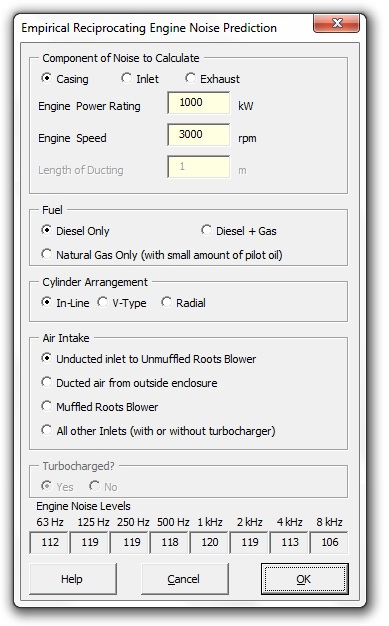### Strutt Help

Empirical Internal Combustion Engine Noise Prediction    1/1

Strutt|Input Data|Empirical|Internal Combustion Engine inserts sound power levels into the active row of the worksheet.The internal combustion engine noise function calculates three components of engine noise.

Exhaust total sound power level is calculated by:

L_W = 120 + 10log_10(kW) - K - (l_(ex)/2), (dB re 10-12 W)

Where K = 0 for an engine with no turbo-charger, K = 6 for an engine with a turbo charger and lex is the length of the exhaust pipe. Octave band levels are then calculated by subtracting the corrections listed in Table 11.19 Bies and Hansen.

Casing total sound power level is calculated by:

L_W = 93 + 10log_10(kW) + A + B + C + D, (dB re 10-12 W)

Where A, B, C and D are correction terms to account for RPM, Fuel, Cylinder Arrangement and Air Intake. These values are sourced from Table 11.21 Bies and Hansen.
Octave band levels are then calculated by subtracting the corrections listed in Table 11.22 Bies and Hansen.

Inlet total sound power level is calculated by:

L_W = 95 + 5log_10(kW) - l/1.8; (dB re 10-12 W)

Where l(m) is the length of the inlet ducting. Octave band levels are then calculated by subtracting the corrections listed in Table 11.23 Bies and Hansen.

References:

• Bies, D., Hansen, C., Engineering Noise Control, pp549 - 551